## A fun introduction to gradient descent optimization for the variational quantum eigensolver algorithm!

This post has 4 sections, so feel free to skip around if you already know some parts and want to save some time (warning: it’ll take a while to get into the quantum part):

Linear regression: building the intuition behind gradient descent

Gradient descent in a nutshell: explained with some math and why it sucks at doing what it’s supposed to do sometimes

Quantum natural gradient (QNG): how we translate natural gradient into its quantum version

The fun part! Applying QNG for VQE for a single qubit case :)

And finally, credits where credits are due + resources I used.

# Linear regression

Remember the last time your math teacher told you to find the line of best fit?

And if you wanted somewhat of an accurate result or just the easier way, you probably picked up your calculator to do it for you.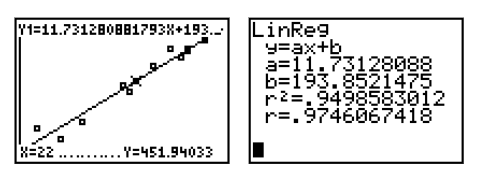Teacher: ‘approximate the line of best fit’. Me: *Uses graphing calculator anyways*

Well, you’re actually doing something called linear regression with your calculator. And that’s just a fancy way of saying fitting a linear equation or a straight line, y = mx + b, to quantify the relationship between the 2 variables in the data.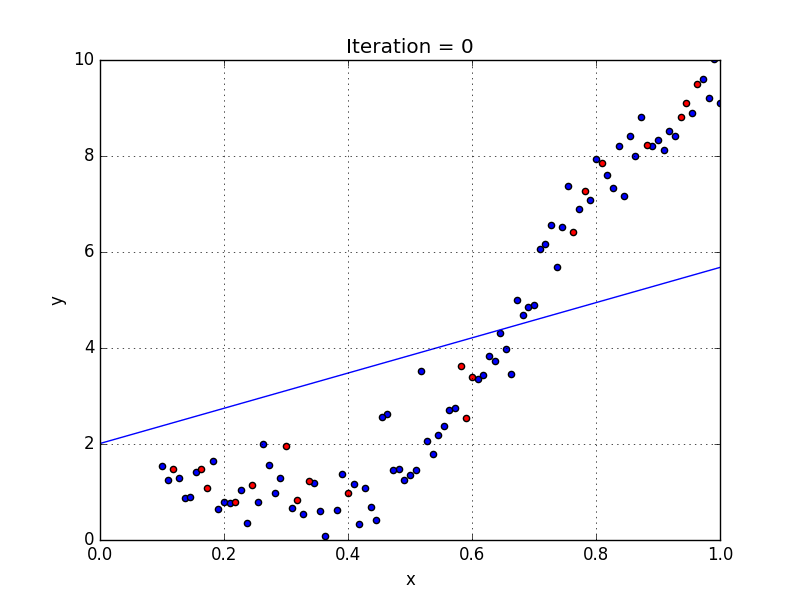That’s what your calculator is doing in ‘finding’ the line of best fit as it ‘plays’ around with m and b values

How the calculator knows when to ‘stop’ (ie, the line of best fit has been found) is by calculating the sum of square differences, also known as the sum of the squared residuals between the observed and predicted values.

## Here’s how you would go about doing that ^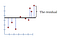Sum of the squared residuals mean you square all the residuals and then add them up.

1) Measure the distance from the line of best fit (the black line) to the data point, square each distance and then add them up. The distance from a line to a datapoint is called a ‘residual’.

2) Each time you shift the line, calculate the sum of the squared residuals

If you plot the sum of the squared residuals against the different rotations, you’ll get something like this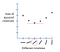Notice that it’s like a parabola if you connected all the points!

The final goal is the find the rotation that gives the smallest sum of squared residuals (the rotation circled red). Here, the derivative (ie, the slope) of the sum of the squares residuals is 0.

While this is a very simplified example, what we just walked through is generally how gradient descent works.

# Gradient descent in a nutshell

## Before we dive in, some real quick definitions:

• Derivative: the ‘rate of change’ or the slope of a function. It shows how fast/slow something is changing at any given point (think tangent line).
• Partial Derivative: When a function is multivariate (meaning it has multiple variables like x and y), we use partial derivatives to get the slope of a function at a given point.

A side tangent — partial derivatives are defined as ‘“a derivative of a function of two or more variables with respect to one variable, the other(s) being treated as constant.”

Example function: (x,y) = y³x + 4x + 5y

∂f/∂x means the partial derivative of f(x,y) with respect to x. where we treat y as constant, so ∂f/∂x = y³ + 4 since 1) (∂f/∂x) of y³x = y³x / x = y³ and 2) (∂f/∂x) of 4x = 4x / x = 4 and 3) (∂f/∂x) of constant 5y = 0.

• Directional Derivative: the vector form of your regular derivation. Example: directional derivative of f wrt (with respect to) a vector x = the dot product of the partial derivative of f wrt x and the normalized form (ie, just take its length and disregard its direction) of the vector x.
• Gradient: a vector pointing in the direction of steepest descent. Its made of partial derivatives of the cost function wrt to each of its variables.

## Gradient descent for more complex functions

Remember the linear regression example? Well, instead of trying to find a line of best fit, you likely have some cost function defined that you are either trying to minimize or maximize (thus, the ‘cost’ on the y-axis because you are trying to optimize its cost).

Here, you can think of the cost as analogous to the line of best fit for a linear function, except its for a most complex function.

The cost function will include some parameters or weights that you are trying to optimize by minimizing them or maximizing them (thus, the ‘weight’ on the x-axis).

Then, you take the derivative of your cost function and you get this nice looking blue parabola where you will start off with some initial value of your parameters or weights. And then you slide down the parabola, weeeeee.

Haha, just kidding. You calculate the gradient at that point (the ‘initial weight’) and you follow your gradient. Then the cost is calculated again is the new weight value, then the gradient… you get it, repeat and rinse until you reach the global cost minimum (or in some cases, the local cost minimum).

And, there’s one more thing you have to take into account. The step size (also known as the learning rate). That’s just how ‘big’ of a step you take each time with your gradient.

The takeaway here is that you’ll need to figure out how to pick a ‘good’ learning rate (sometimes, it’s just trial and error). If each ‘step’ you take is too big, you end up zig nagging. But if each ‘step’ is too small, it will take forever to reach the local/global optimum. TL;DR if your learning rate sucks, your gradient descent algorithm isn’t going to perform very well. Such a minor detail but also a party pooper.

Soooo, when we get into multivariable functions, the contoured landscape that your gradient descent algorithm needs to navigate becomes a lot more complex. Something like this:

## ^, but expressed mathematically

The steps you would take as follows:

1. Pick an initial value for x (ie, the weights) — usually, randomly
2. Calculate the gradient of the cost function wrt to the parameters (x)

3. Adjust the parameters x, where a is the learning rate / step size, such that:

And then you just repeat steps 2 and 3 until you get to a local or global optimum where the cost value is ‘optimized’ (minimum or maximum but usually a minimum).

Congrats! You now know how gradient descent works + the math behind it!

## What’s the big deal?

Well, despite the endless variations of gradient descent algorithms that we’ve come up with (ie, stochastic gradient descent, batch gradient descent…) there’s been a huge problem within the field known as barren plateaus.

Pretty much this but as the landscape that your gradient descent algorithm needs to navigate. As you can imagine, your algorithm may end up in one of these areas where the landscape is entirely ‘flat’ around that point. The result is you get ‘stuck’ and end up going nowhere.

Alternatively, another problem is that the algorithm gets ‘stuck’ in a local minimum when you’re really trying to find the global minimum.

The implication here is that gradient descent algorithms, which are an integral part to machine learning algorithms (which have endless applications) don’t perform as well as they should be performing.

We have some more pitfalls (no pun intended) as follows:

• The learning rate / step size is often arbitrarily determined. And since the gradient is the first derivative of the loss function and by definition, it only knows the slope at the point at which it was calculated. Thus, it can only ‘see’ the slope at the point, but not over a segment. So, how does it know if it should take a smaller or larger step? It simply doesn’t. Well, unless you take the 2nd order derivative — but that’s often complex and computationally expensive + inefficient.
• This update also treats all the weights / parameters equally (they are all scaled by the learning rate). In reality, we might have some parameters that are more important than others. Thus, by restricting the update for such important parameters, we take longer than necessary for the algorithm to converge (reach the optimum).

This is where *quantum* comes in to save the day (literally and figuratively).

As always, there’s the classical version that needs to come first haha. So, we’ll need to go through the good ol’ regular natural gradient before.

Regular gradient descent works by updating parameters using the Euclidean metric, which in a sense is ‘blind’ to the geometry of the parameter space. In comparison, the classical natural gradient descent takes advantage of the geometry of this parameter space…

## Natural gradient descent: KL divergence

Here, we don’t fix the learning rate at a constant because there is *surprise* another way to ‘prevent’ the algorithm from blindly jumping all over the place. Instead of fixing the euclidean distance each parameter moves (ie, the distance in the parameter space), we fix these distances on the output distribution space.

So, instead of changing the parameters within some defined distance from the learning rate, we limit the output distribution so that it is some distance from the distribution of the previous step.

Though, the catch here is that this isn’t really a ‘distance’. So, we ‘measure’ this difference between the 2 distributions using something called the Kullback-Liebler Divergence (KL Divergence).

Something like this where the KL divergence is the red line.

Notice that while the overlaps between the 2 Gaussians (the red and orange bell-curved graphs on the left vs on the right) look very different, the red line is the same length (ie, the Euclidean distance).

There is much greater overlap on the left on the right, meaning the distribution on the right underwent a much greater ‘transformation’. However, the ‘means’ have actually been shifted the same amount (ie, the KL divergence).

In a nutshell, we are no longer moving in the Eulicdean parameter space, but instead, the distribution space with KL divergence as the metric, which is invariant to the parameterization of the distribution.

So with this little bit of extra information, we get to ‘navigate’ the parameter space a lot more efficiently.

## Natural gradient descent: Fisher information matrix

This matrix allows us to work in the distribution space.

I’ll skip over the details which you learn more about here (and I also won't go into the nuances of the math behind how KL divergence translates into the Fisher matrix but it, in essence, the Fisher Information Matrix defines the local curvature in distribution space for which KL-divergence is the metric). [source]

If we include this matrix in the regular gradient descent formal, we get something like this:

So, your gradient descent steps look a little bit different, with 2 changes:

• The learning rate (α) has been replaced with ηₜ, because it may change each with each iteration of the algorithm
• We have an additional term, which is the inverse of the Fisher matrix (F(θₜ)^-1), is added to the partial derivative we had for the original gradient.

So why hasn’t this been used if it’s so great?

Well, in the world of classical computing, the calculation of the Fisher matrix and it’s inverse becomes really computationally hard (and expensive) to calculate as the number of parameters grows.

And in your typical neural networks, there are lots of parameters. So in the meantime, your genius researchers deep learning researchers have figured out a bunch of other gradient updates that are better than natural gradient, like momentum and adam (which are variations of stochastic gradient descent).

But, if we translate this over into the quantum world, the complexity of this problem no longer scales as fast!

## The quantum version of natural gradient (QNG)

QNG is basically the same algorithm as natural gradient but since we’ve moved into the quantum world, we need to appropriately adapt our metric.

We use the Fubini-Study metric (also known as the quantum fisher metric), which does the same things as KL-divergence in terms of defining the ‘distance’ between two output distributions.

• |φ(θ)⟩ is the initial ansatz and ∂|φ(θ)⟩/∂θ_i is the partial derivative of this wrt to θ_i (which is our parameter).
• The first term of the metric is the real part of the inner product of the bra of the partial derivative of the initial ansatz wrt to θ_i times the ket of the initial ansatz wrt to θ_j.
• The second term of the metric is the dot product of 2 inner products, both of which contain the partial derivative of the initial ansatz wrt to θ_i or θ_j.

So, by playing around in the distribution space with this non-Euclidean metric, we get to take into account (and thus, take advantage) of the geometric properties of parameterized quantum states that are otherwise ‘ignored’ by the regularEuclidean metric.

Here’s exactly how:

Around singularities in the parameter space, where the ‘volume’ of the metric becomes really small, the natural gradient takes this into account and drives the parameter point faster than the ordinary gradient.

Specifically, the determinant of the Fubini-Study metric (F) is 0, ie set(F) = 0, at these ‘singularities’ whereas other metrics fail to capture this geometry of the parameter space.

When the determinant of a matrix (which can be thought of a transformation of the ‘space’) is 0, the ‘space’ squishes into a line or a point, which is why these are called singularities.

Thus, if the matrix can take into account these points, then they can actively avoid them.

In fact, this is exactly what QNG does.

The red dotted line represented where these singularities are in the parameter space defined by 2 parameters (θ_1 and θ_2) and you can see that QNG actively ‘avoids’ passing through these points while other algorithms pass through them.

This is because the determinant of the Fubini-Study metric is 0 at these singularities, while it is not for the other algorithms, meaning they cannot ‘capture’ this extra information about the parameter space.

And that’s precisely why QNG can outperform classical forms of gradient descent!

# The fun part: 1 qubit example

#1: Install everything: you’ll need 1) pennylane 2) tensorflow 3) QuTip

#2: Next, we’ll import everything that we’ll need to use:

`import pennylane as qml from pennylane import numpy as np from pennylane import tensorflow as tf from pennylane.qnodes import PassthruQNode  import qutip as qt from qutip import Bloch, basis`

#3: Create a quantum device with 1 qubit and define our circuit. Using PassthruQNode function, we get a differentiable quantum state that will allow us to get the state vector at each iteration by ‘measuring’ the circuit.

`dev = qml.device('default.qubit', wires=1)  def circuit(params, wires=0):        qml.RX(params, wires=wires)        qml.RY(params, wires=wires)  qnode = PassthruQNode(circuit, dev)`

Notice the circuit has 2 parameters, which is taken into account when we define our Hamiltonian (which has 2 terms).

#4: Define the hamiltonian using a list of observables and then initialize the circuit parameters as a random array of size 2. For more details on how the cost function works, see here.

`coeffs = [1, 1]obs = [qml.PauliX(0), qml.PauliZ(0)]H = qml.Hamiltonian(coeffs, obs)cost_fn = qml.VQECost(circuit, H, dev)init_params = np.random.uniform(low=0, high=2*np.pi, size=2)`

#5: Finally, we optimize the cost function over the two parameters θ_1, params, and θ_2, params and then ‘measure’ the circuit each time to yield the statevector at each step.

`step_size = 0.01max_iterations = 500conv_tol = 1e-06print_freq = 20params = init_paramsprev_energy = cost_fn(params)qng_energies_block = []all_states = []for n in range(max_iterations):        grad_cost = qml.grad(cost_fn, argnum=)    grad_at_point = [float(i) for i in grad_cost(params)]         params = params - step_size * np.dot(                np.linalg.pinv(qnodes.metric_tensor([params])),                 grad_at_point)    energy = cost_fn(params)    qng_energies_block.append(energy)        conv = np.abs(energy - prev_energy)    state_step = dev._state    all_states.append(state_step)    if n % print_freq == 0:        print('Iteration = {:}'.format(n) ,'Energy = {:.8f} Ha,'.format(energy), 'Convergence parameter = {'              ':.8f} Ha'.format(conv), "State", state_step)    if conv <= conv_tol:        qng_block_steps = n        break    prev_energy = energy    state_final = dev._stateprint()print('Final value of the ground-state energy = {:.8f} Ha'.format(energy))print()print("Final state", state_final)print()print('Number of iterations = ', n)`

The output looks something like this:

`Iteration = 0 Energy = 0.15600095 Ha, Convergence parameter = 0.07461159 Ha State [0.46631216+0.49735071j 0.63331982-0.36619836j] Iteration = 20 Energy = -1.09798577 Ha, Convergence parameter = 0.03398730 Ha State [-0.31035061+0.31209166j  0.8913321 +0.10866638j] Iteration = 40 Energy = -1.37976149 Ha, Convergence parameter = 0.00419391 Ha State [-0.36584753+0.10158901j  0.92423926+0.04021262j]Iteration = 60 Energy = -1.41081958 Ha, Convergence parameter = 0.00041861 Ha State [-0.37795909+0.0317502j   0.9251868 +0.01297065j] Iteration = 80 Energy = -1.41388272 Ha, Convergence parameter = 0.00004086 Ha State [-0.38125941+0.00989945j  0.92440608+0.0040829j ] Iteration = 100 Energy = -1.41418135 Ha, Convergence parameter = 0.00000398 Ha State [-0.38224392+0.00308785j  0.92405542+0.00127732j]  Final value of the ground-state energy = -1.41420560 Ha  Final state [-0.38245235+0.00162716j  0.92397354+0.00067352j]  Number of iterations =  112`

#6: To visualize the results on a bloch sphere, we first need to convert all the statevectors that we measured into QObjects that QuTip will recognize. We’ll only plot every 10th statevector for the sake of simplicity.

`# Get every 10th statevector and convert into Qobj #plot_states = []lst = all_states[::10]for i in range(len(lst)):  psi = lst[i]  psi = psi/np.linalg.norm(psi)  coords = [qt.Qobj(psi)]  plot_states.append(coords)# Convert Qobj into x, y, z coordinates #from qutip.expect import expectfrom qutip.operators import sigmax, sigmay, sigmazcoords_x = []coords_y = []coords_z = []for qobj in plot_states:    st = qobj    x = expect(sigmax(), st)    y = expect(sigmay(), st)    z = expect(sigmaz(), st)    for i in range(len(x)):      x_list = x[i]      coords_x.append(x_list)      y_list = y[i]      coords_y.append(y_list)      z_list = z[i]      coords_z.append(z_list)print(coords_x)print(coords_y)print(coords_z)`

And then we plot it…

`import matplotlib as mplimport matplotlib.pyplot as pltfrom matplotlib import cmnrm = mpl.colors.Normalize(0, len(coords_x))colors = cm.jet(nrm(range(len(coords_x))))b = Bloch()b.sphere_alpha = 0.1b.figsize = [8,8]b.point_color = list(colors)b.point_marker = 'o'b.point_size = b.add_states(plot_states, 'point')b.add_points([coords_x, coords_y, coords_z], 'l')b.show()`

And then we get this beautiful result!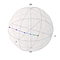You can see the ‘progression’ of the state vector as it approaches the ground state (the red point) given a random initialization (the purple point)

Success 🎉!! You made it all the way to the end.

Personally, I can’t wait to see the future of variational algorithms and their applications in solving complex computational problems!

## Thank you’s + acknowledgements

Huge huge shoutout to mentor, Hannah Sim and partner Lana Bozanic for the fun times + immense learnings while working on this project. We’ve dove deep into the world of quantum natural gradient descent while working through papers to implement examples and developing a code base for more complex VQE problems that can use QNG. Stay tuned for more!

Another special thank you to the amazing people at the Quantum Open Source Foundation (QOSF) for running the quantum mentorship program. This wouldn’t have happened with them!

And credits to Xanadu AI’s Pennylane developers for the original code base + the awesome documentation!

## References

• Stokes et al (2019): Quantum Natural Gradient [here]
• Yamamoto et al (2019): On Natural Gradient for Variational Quantum Eigenoslver [here]
• Original pennylane QNG tutorial [here] and VQE tutorial [here], both of which were used as codebases
• Blog post on KL Divergence and the Fisher Information matrix [here]

Thanks for reading! I love all things quantum ⚛️ +bio 🧬 and also occasionally write to document my learning.

If you’re interested in following me on my journey, connect with me on Linkedin, follow me on Twitter, or subscribe to my monthly newsletters!

I hope you learned something new!

Quantum computing + all things bio! http://lzylili.com/

## More from Maggie Li

Quantum computing + all things bio! http://lzylili.com/